Question

Modern Physics

a) You measure the energies of the 1000 ideal gas (non-interacting) molecules in this 1-D vessel and find that 500 have the ground state energy, 300 have the 1st excited state energy and 200 have the 2nd excited state energy. Write y for a molecule in this gas consistent with these measurements.

b) Use the equation for expectation values to calculate the average energy of molecules in this gas. Is this consistent with calculating the average energy directly from the information given in part a?

c) Immediately after measuring that a molecule is in the 1st excited state you measure its momentum. What are the possible values you measure and their probabilities?

d) You adiabatically (no change in populations of the different energy levels, which is equivalent to no heat exchange with the surroundings) compress this gas so that the cylinder now has width L/3. What is the change in total energy of the system? How much work was done on the gas? Does the temperature change? Does the amplitude of p change? Explain how these results are consistent with what you learn in the thermodynamics part of PChem.Verified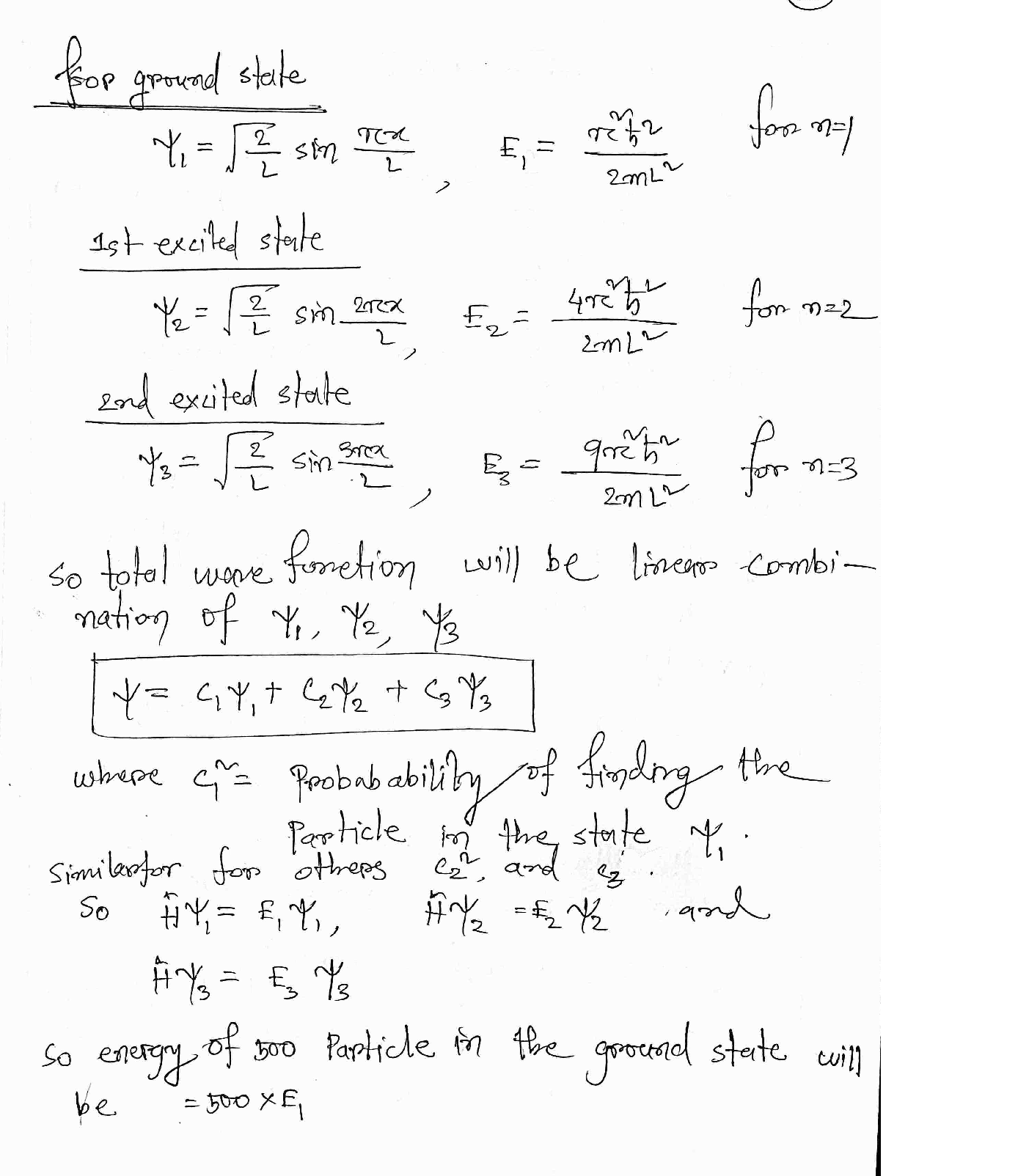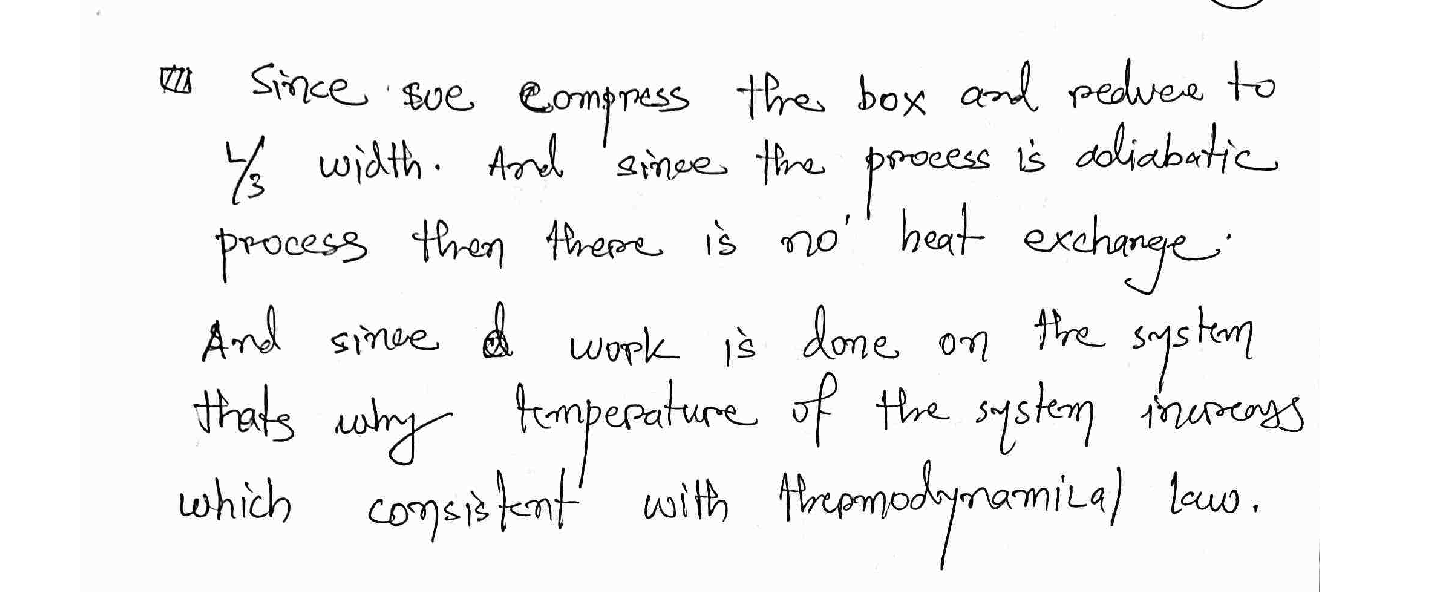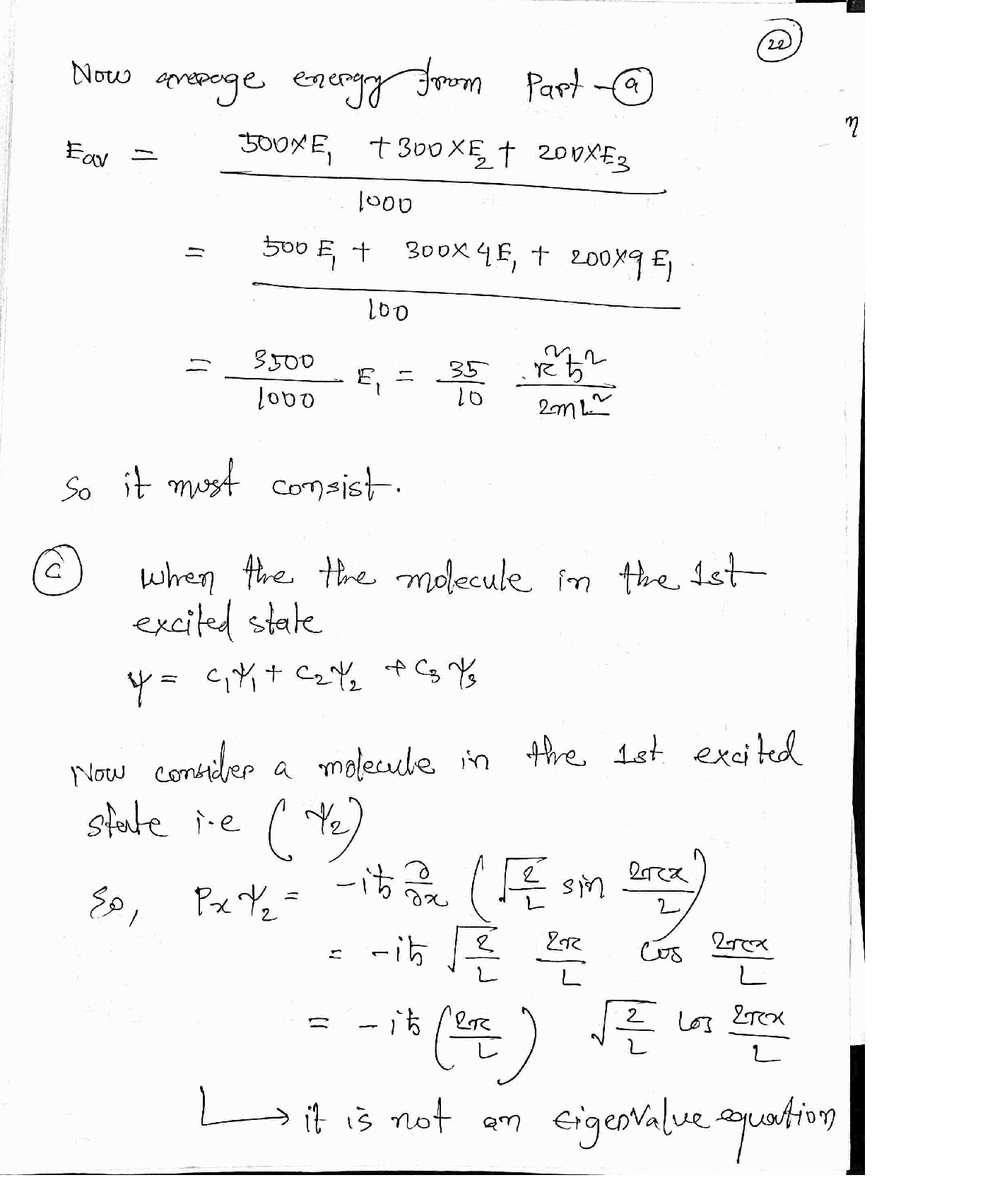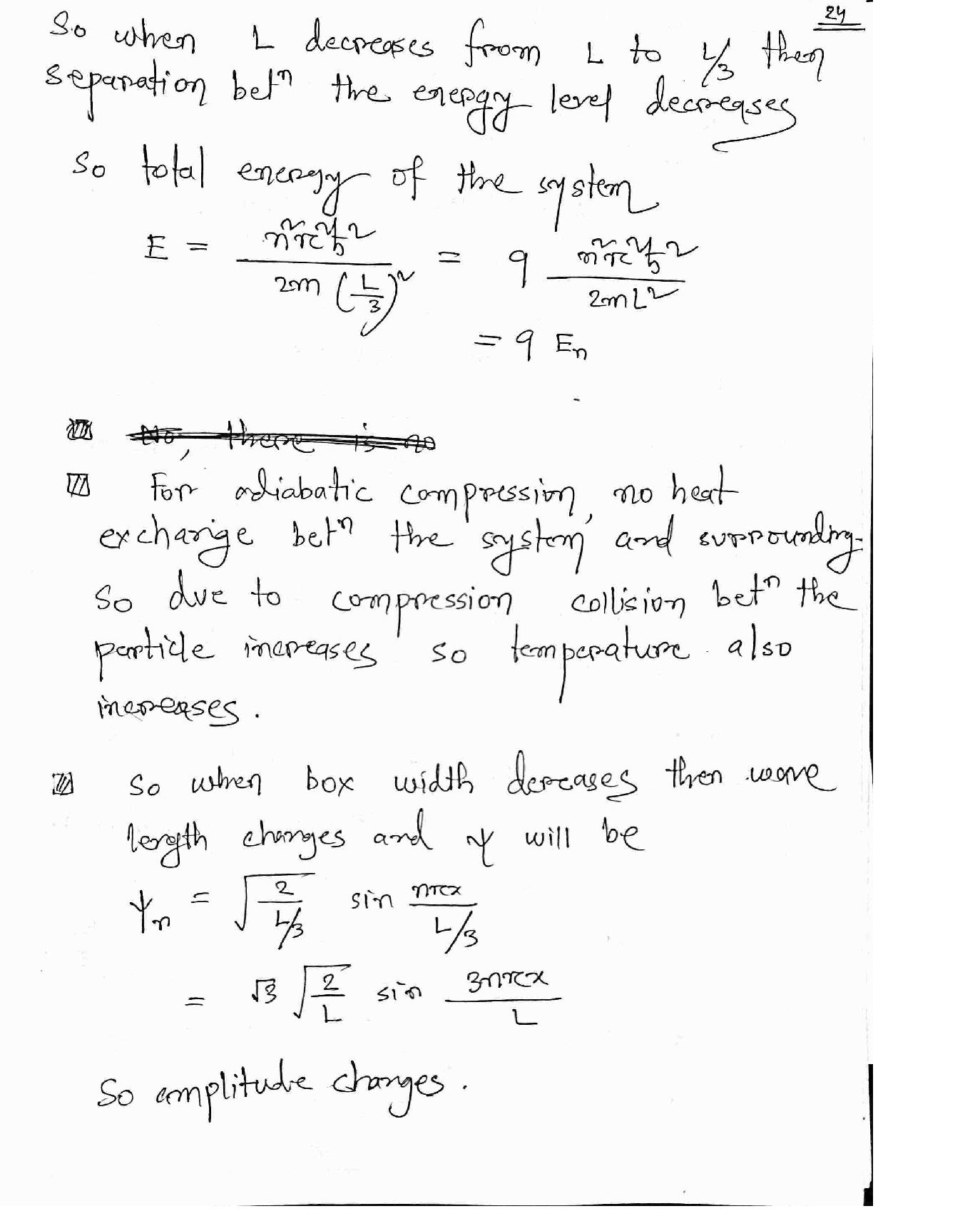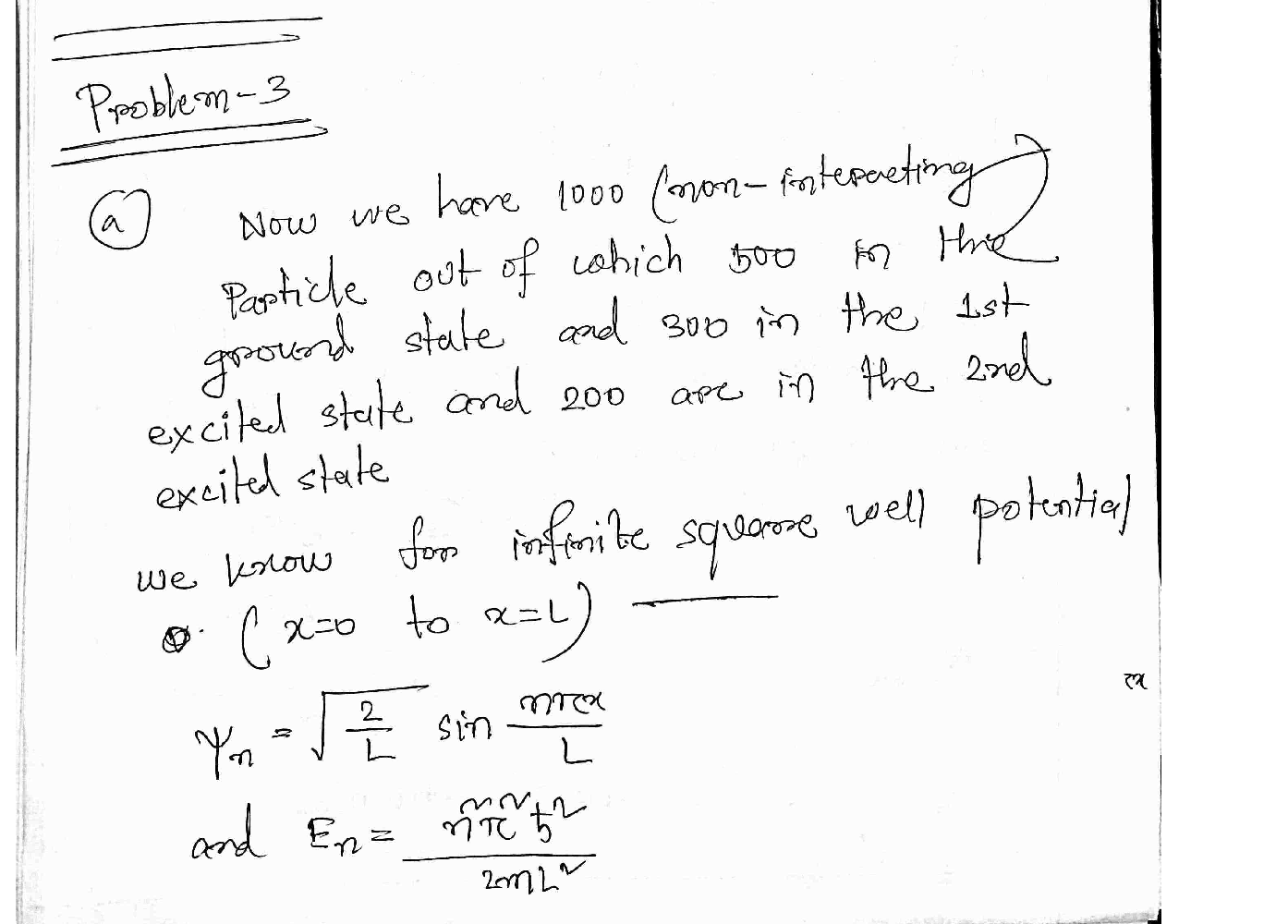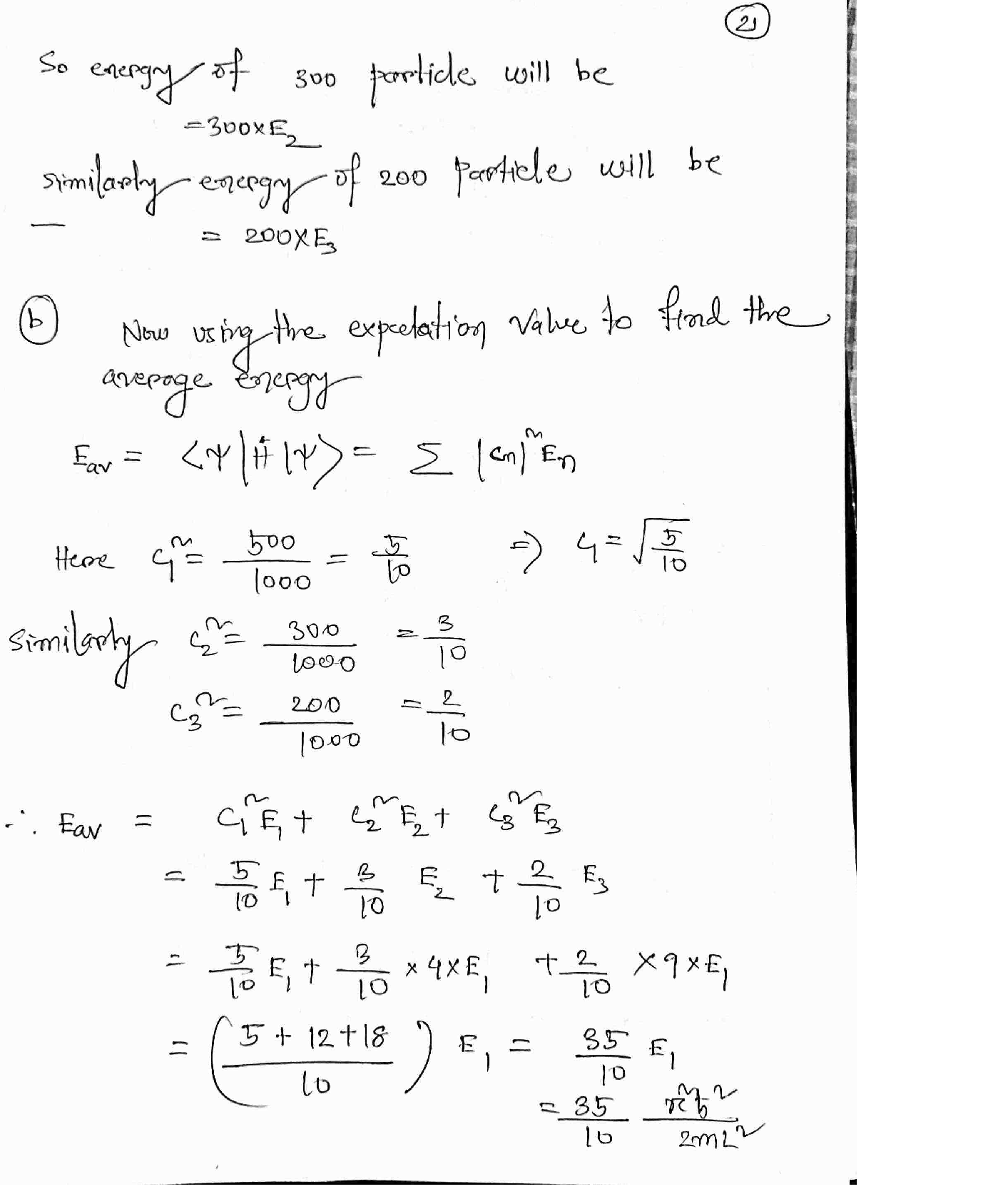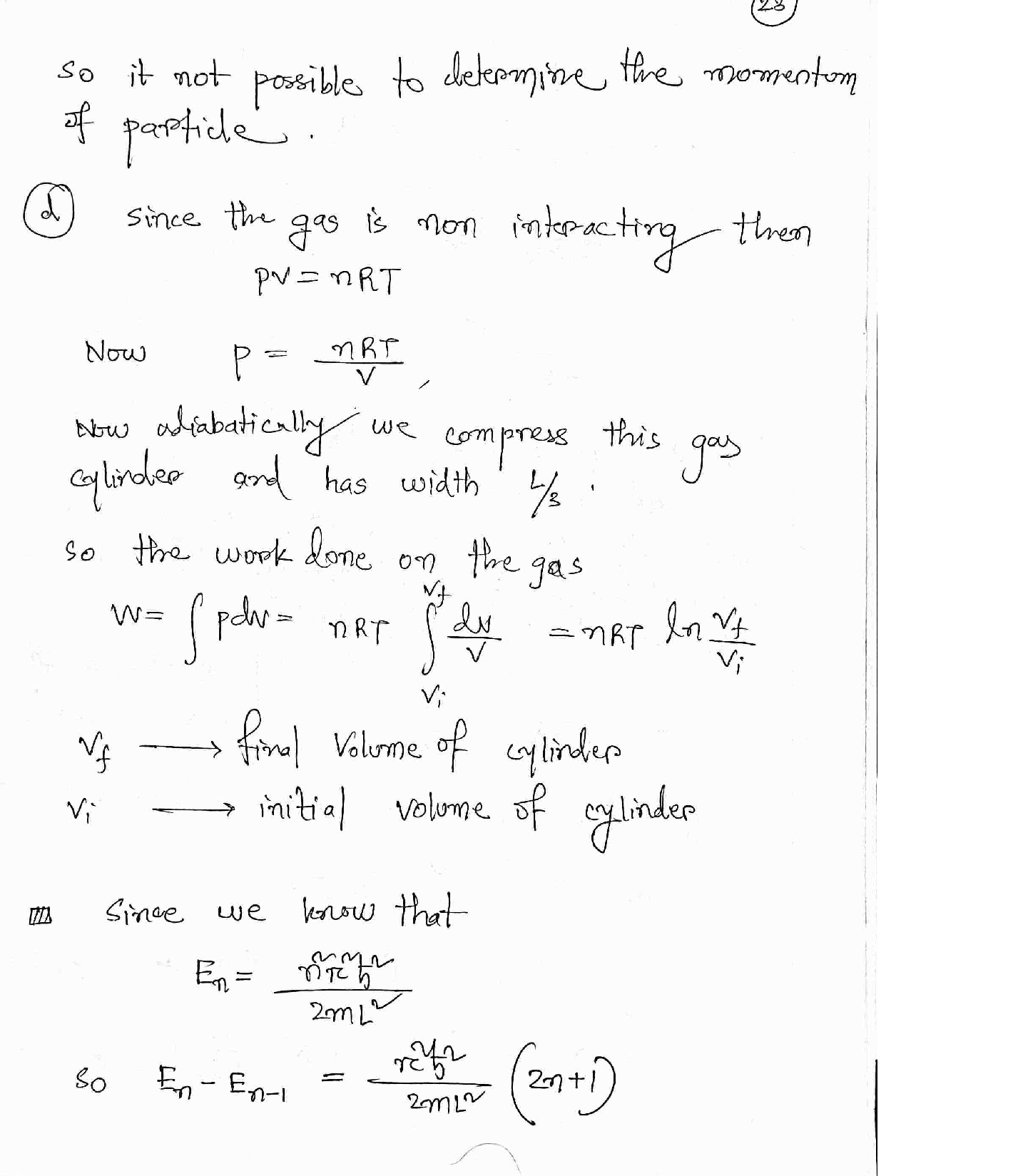### Question 44658Modern Physics

Question 5: A spaceman goes out on a trip. First, he travels at 1/3 the speed of light in one direction. Then he comes back home at 2/3 of the speed of light. On Earth 9 years have passed,by how much has the spaceman aged? (Make sure you draw a space-time diagram).

### Question 44657Modern Physics

Question 4: The typical pole for pole vaulting is 17 feet long. How fast would a pole vaulter have to run (in the direction of the pole) in order for a spectator sitting in the stands to see it 15feet long?

### Question 44656Modern Physics

Question 3: If you weigh 150 lb in Chicago, how much do you weigh on top of mount Everest?(mount Everest is 8,848 m high).

### Question 44655Modern Physics

Question 2: As a result of an explosion a stationary object breaks into three pieces. One piece flies east, one west and one north.
Explain why this is impossible.
Now, there are only two pieces; one flies east the other west. One piece weighs three times as much as the other. What is the ratio of the kinetic energies of the two pieces?

### Question 44654Modern Physics

Question 1: The international Space Station (ISS) orbits the Earth once every 90 mins at an-altitude of 409 km. How high would it have to be in order to be to be in geosynchronous orbit?(make sure you show how you worked it out).

### Question 44179Modern Physics

Question 6: A "star" made of ideal gas radiates away 10% of its energy while remaining in hydrostatic balance. Assuming that there are no nuclear reactions
Does the radius of the "star" increases or decreases? By how much?
Does the (average) temperature of the "star" increases or decreases? By how much?

### Question 44178Modern Physics

5: There are two stars, one has twice the luminosity of the other and half the radius.
What is the ratio of their temperatures?
What is the ratio of their (apparent) brightness if the more luminous one is 100 times more distant than the other.

### Question 44177Modern Physics

Is that true?
Question 4: According to general relativity people who live in hi-rise buildings age at differentrates depending on their floor.
Which floor ages fastest?

### Question 44176Modern Physics

Question 3: Consider two time-like events P and Q. P is at the space-time origin P=(0,0); Q is at the space origin but one second later Q=(0,1).
Show that the order of events is preserved by all observers travelling at less than the speed of light.
Now events P and Q are space-like. P is still at the origin, but Q is simultaneous with P but one meter to the right 0=(1.0)
.Show that the order of events is not preserved by observers travelling at less than the speed of light.

### Question 43068Modern Physics

6. (15 points total) The main composition of main sequence stars are known to be hydrogen. Hydrogenundergoes nuclear fusion reaction in the inner core of the star and generate a complete spectrum ofelectromagnetic waves. However, before the electromagnetic waves leave the surface of the stars, thehydrogen at the outer core of the star would absorb certain wavelengths of the electromagnetic waves andbecome excited. Therefore, we should expect absorption of light at wavelength of 656.3 nm, 486.1 nm, etc.,for all stars, indicating the presence of hydrogen. However, as you analyze the spectrum of a star, youobserved absorption line at 662.9 nm, 491.0 nm, etc. All wavelengths of the absorption lines corresponding tothe Hydrogen Balmer series are shifted up.Scanned with CamScanner
6a. Is the star moving towards or away from the Earth? Explain briefly.CamScanner
To find the speed is the star moving relative to the Earth, following these steps:
i. Find the corresponding frequencies of the 656.3 and 662.9 nm lines in Hz. Determine which one is fL and which one is fS.Scanned
ii. Relate fL and fS with c and v (relative velocity between the star and the Earth).
ii. Find v in m/s.
6c. To verify this discovery, you borrowed and installed a near infrared sensor on your telescope. Use the Rydberg's formula to calculate the infrared wavelength for the n: 4 -> 3 transition before Doppler shift in um.Scanned with CamScanner
6d. Then apply the Doppler's effect formula for electromagnetic waves to calculate the real wavelength you would observe in um by considering the star's motion.Scanned with CamScanner
6e. In general, the absorption spectrum of stars are redshifted (which means the wavelength of the absorptionlines are all longer than expected). Is this an indication that the universe is expanding or contracting?Scanned with CamScanner
O ExpandingO Contracting

### Submit query